### Browse Departments

##### Pottery Illustrated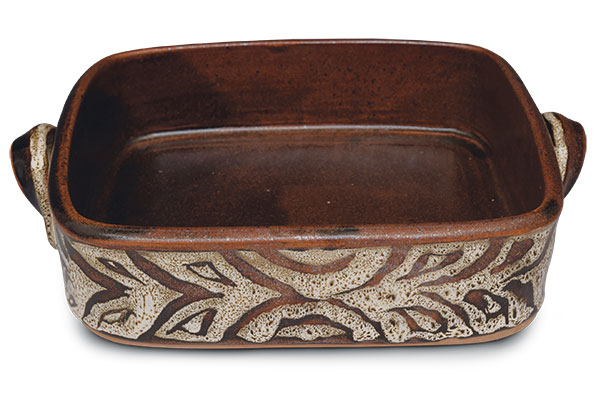Maybe you are among the many potters who are intimidated by math—you’re an artist, you’re intuitive, you naturally see proportion and balance, so who needs math? But since we work with a material that shrinks, math can be critically important to making certain functional wares. If you want to make a standard-sized dinner plate, a baking dish, or a mug that fits under your coffee maker, you’ll need to calculate shrinkage.

### Calculating Shrinkage

Most stoneware clay bodies shrink around 12.5%. If you have LidMaster calipers, they even have a 12.5% shrinkage adjustment built in. This is an easy shrinkage rate to visualize as it works out to a 1/8 rate of change. This means if you make something 8 inches high in wet clay, it will shrink to 7 inches high.

If you use a commercial clay body, you can find out the shrinkage rate from the manufacturer, but if you use your own recipe, you will need to run shrinkage tests by measuring and marking a 10-inch line on a rectangular slab of wet clay, then measuring the length of that line after firing to the maturation temperature to find the shrinkage rate. For the purposes of this article, I’m going to go with 12.5% as the shrinkage rate.

Let’s suppose you want to make a standard-sized dinner plate. First of all, what is standard? There’s not actually a definite answer to this question because the standard keeps changing. The standard 50 years ago was 9 inches, then it became 10 inches, and now it is 11–12 inches (clearly our dinner plates are expanding along with our waistlines). So, pick your preferred standard size. If you wanted to make a 7-inch diameter plate, the answer is easy: throw it to 8 inches in diameter. If you want to make an 11-inch plate, how big do you have to throw it (1)? There are a couple of approaches to take. If your clay shrinks 12.5%, or 1/8, one answer is to divide the desired fired dimension by 7 and multiply by 8 to find the wet dimension.The wet clay will shrink to 7/8 of its size, so you make it 8/7 as big as you want it to end up. In this case, 11 divided by 7 is about 1.57. Multiply that by 8 and you have 12.57. Even easier, simply add 1.57 to 11 to get 12.57. Okay, where is 12.57 on a ruler? If you need your measurement to be exact, you might want to switch to centimeters. There are 2.54 cm in 1 inch; 2.54×11 is 27.94 cm. Now, divide by 7 and multiply by 8 and you get 31.93 cm.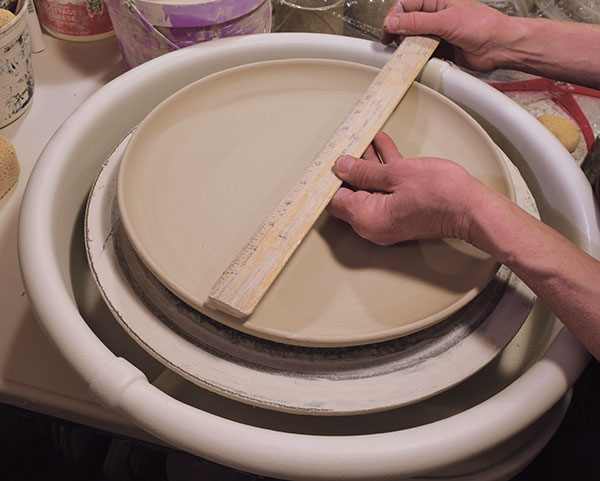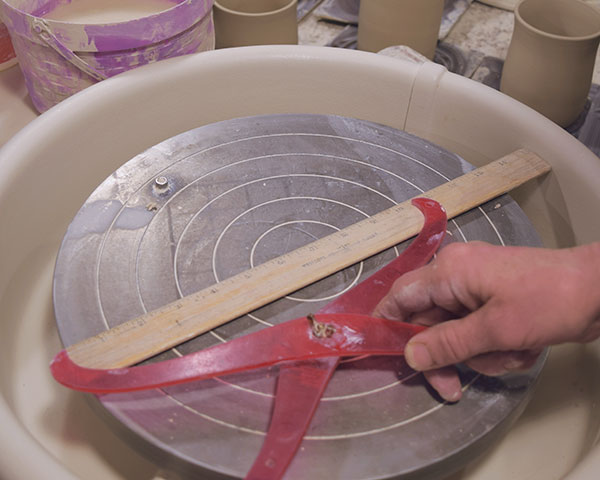A metric ruler has big lines for every centimeter and smaller lines for every millimeter. So, 31.93 cm is 31 cm plus 9 mm. The extra 0.3 mm is somewhere between two tiny marks and it’s very unlikely you need to worry about that 0.3 mm for a dinner plate.

If your clay shrinks a different amount—say you work with low-fire clay and it shrinks 10%—then the shrinkage is a 1⁄10 rate of change, shrinking to ⁹⁄10 of its size. In this case, you’ll divide your fired size by 9 and multiply by 10 for your wet size. If your clay shrinks 12% instead of 12.5%, you need to divide your fired size by 88 (100 minus 12) and multiply by 100 for the wet size.### A Looser Approach

My usual approach is a little looser and more intuitive. Working with a 12.5% shrinkage rate again, I know that 12 inches will shrink 1½ inches (1/8 of 12 is 1½). So if I throw a 12-inch plate, I know it will end up 10½ inches in diameter, half an inch too small. So, what if I added that ½ inch and throw it to 12½ inches? Well, that ½ inch would also shrink 1/8, which is 1/16 inch, so I have to make it 1/16 of an inch larger, meaning I’d throw a plate that was 12½ inches plus 1/16 of an inch. The little marks on a ruler are 1/16 of an inch, so throw it to one little mark past 12½ inches.

### A Few More Examples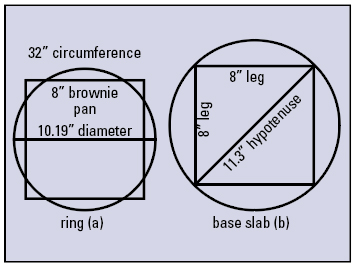If you want a 9-inch pie plate, throw it a tiny bit bigger than 10¼ inches. Why? Because 10 inches will shrink 1¼ inch, so if you make it exactly 10¼ inches, that extra ¼ inch will make it shrink a tiny bit more. If you want a mug to be 3½ inches high, that’s easy—make it 4 inches tall and it will shrink ½ inch. Want it 4 inches tall? You have to add half an inch plus a tiny bit: make it 4½ plus 1/16 inch tall. I find this easier to visualize than dividing 4 by 7 and multiplying by 8 (the answer is 4.57, but try finding that on a ruler). If you have calipers, you can use them instead of a ruler to measure your freshly thrown ware. For a pasta bowl that needs to be 9 inches in diameter, I use a ruler to set my calipers to 10 3/16 inches (2), and then measure a freshly thrown bowl with the calipers.

If you have LidMaster calipers, you can avoid math altogether. Simply take out the screw in the middle and reset the calipers to the 12.5% shrinkage setting. Now, open it up so the narrower end measures your desired fired size. The wider end will now be set to the exact measurement for the wet clay. (The standard sized LidMaster only stretches to 12 inches though, so you’d have to have the large size for the dinner plate.) Even if I use calipers this way, I still like to calculate the correct measurement, just to make sure I really did set them correctly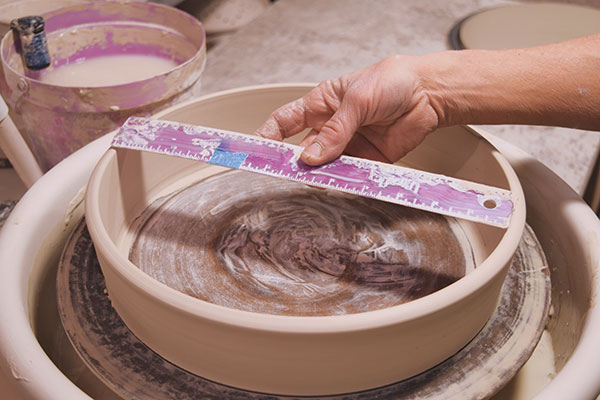### Solving for a Square or Rectangle

Here’s a slightly more complex problem, and one that you can’t entirely solve by resetting your calipers. I like to make a square or rectangular baking dish by throwing a ring without a bottom, altering it into a square, and then attaching it to a slab. If I want to make an 8×8-inch brownie pan, how big of a ring do I throw? I need to figure out the circumference of the ring first—that’s the distance around it. Since I’m going to take a circle and turn it into a square, the sides of the square will add up to the circumference of the circle. So, if I want a square that is 8 inches on each side, the circle will have a circumference of 4×8, or 32 inches. That is after shrinkage, of course. But wait, I’m not going to wrap a measuring tape around the ring as I’m throwing it to figure out the circumference. I need to know what diameter to throw the ring. The relationship between diameter and circumference involves the number pi: the circumference of a circle is pi times the diameter. To figure out the diameter (3a), I need to divide the circumference by pi. Pi is 3.14—well, it’s a longer number than that, but 3.14 is good enough. (In fact, dividing by 3 is usually good enough for most projects.) So, 32 divided by 3.14 is 10.19.But we haven’t accounted for shrinkage yet. I need to divide 10.19 by 7 and multiply by 8 to find the wet diameter of the ring I need to throw.The answer is 11.646. In other words, it’s somewhere between 11½ and 11¾. In fact, it’s really close to 11⅝ inches, which is a line that is marked on most rulers. Set your calipers to 11⅝ inches and throw a ring to that diameter, or just use a ruler to measure across the ring. Or, if you have calipers with a 12.5% shrinkage adjustment, use them—set the narrow end to just under 10¼ inches and the wide end will be about 11 ⅝ inches. Or, if you have LidMaster calipers with a 12.5% shrinkage adjustment, use them—move the screw in the middle to the second set of holes, then set the narrow end to just under 10¼ inches and the wide end will be about 11⅝ inches.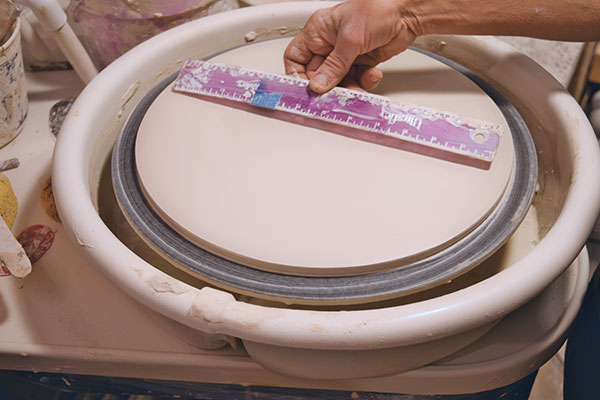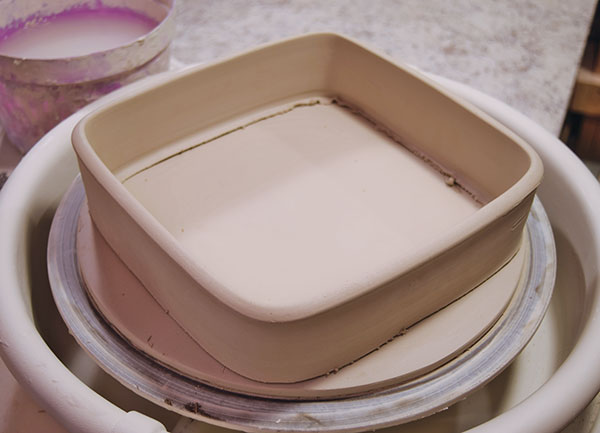Once you throw and measure your ring (4), let it stiffen up just a bit, then alter it into a square. To add one more twist, I like to throw slabs on the wheel to make the base. This saves clay because I can calculate the exact size to throw my slab so that I won’t have to cut much off. The diameter of the slab will need to be the diagonal of the 8-inch brownie pan, so I’ll need to figure out that measurement before shrinkage. From high school geometry class you might remember the Pythagorean theorem (a2+b2=c2): the square of the hypotenuse of a right triangle is the sum of the squares of the side of the triangle. The triangle in question has two 8-inch sides and the hypotenuse is the diagonal across the brownie pan (see 3b). The square of 8 is 64. Multiply 64×2 and you get 128. I know that the square of 11 is 121, so therefore the hypotenuse is a bit more than 11, somewhere in the ball park of 11.3 (11.3 squared is 127.69, really close to 128). That’s before shrinkage; so divide 11.3 by 7 and multiply by 8 and you have 12.91. If you throw a slab about 13 inches in diameter (5), you’ll be able to attach your altered ring to it (6) and when you cut off the edges, you won’t be recycling too much clay.

If you love math, you’ll love solving problems like throwing a brownie pan. If you are intimidated by math, I hope you’ll at least feel confident enough now that you can make a dinner plate or a pie pan to standard measurements. Sooner or later, someone is bound to ask you to make something in a particular size and if you know to divide by 7 and multiply by 8, if your clay’s shrinkage is close to 12.5%, that will usually be good enough.

Sumi von Dassow is an artist, instructor, and regular contributor to Pottery Making Illustrated. She lives in Golden, Colorado. Check out Sumi’s book, In the Potter’s Kitchen, published by The American Ceramic Society and available in the Ceramic Arts Network Shop, https://mycan.ceramicartsnetwork.org/s/product-details?id=a1B3u000009udqFEAQ.

Topics: